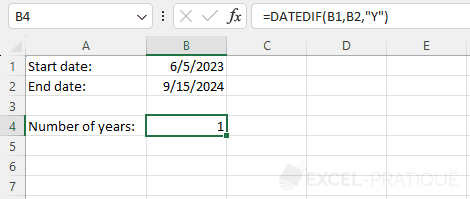# Excel Function: DATEDIF

The DATEDIF function calculates the number of days, months or years between two dates.

Usage:

`=DATEDIF(start_date, end_date, unit)`

## Number of days between 2 dates

To calculate the number of days between two dates (for example "06/05/2023" and "09/15/2024"), enter these dates into the DATEDIF function along with the unit "D" for the number of days:

``=DATEDIF("06/05/2023","09/15/2024","D")``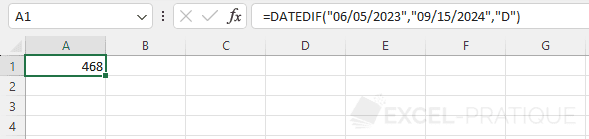If the dates are in cells, enter:

``=DATEDIF(B1,B2,"D")``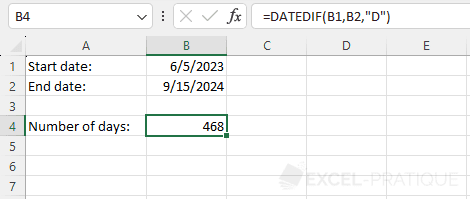Note that in this case you could also simply enter:

``=B2-B1``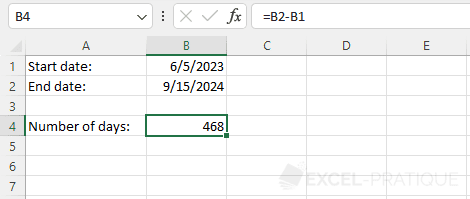## Number of months between 2 dates

To calculate the number of full months between 2 dates, enter the unit "M":

``=DATEDIF(B1,B2,"M")``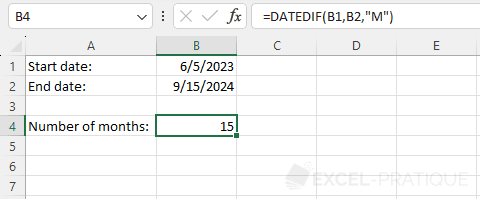## Number of years between 2 dates

To calculate the number of full years between 2 dates, enter the unit "Y":

``=DATEDIF(B1,B2,"Y")``